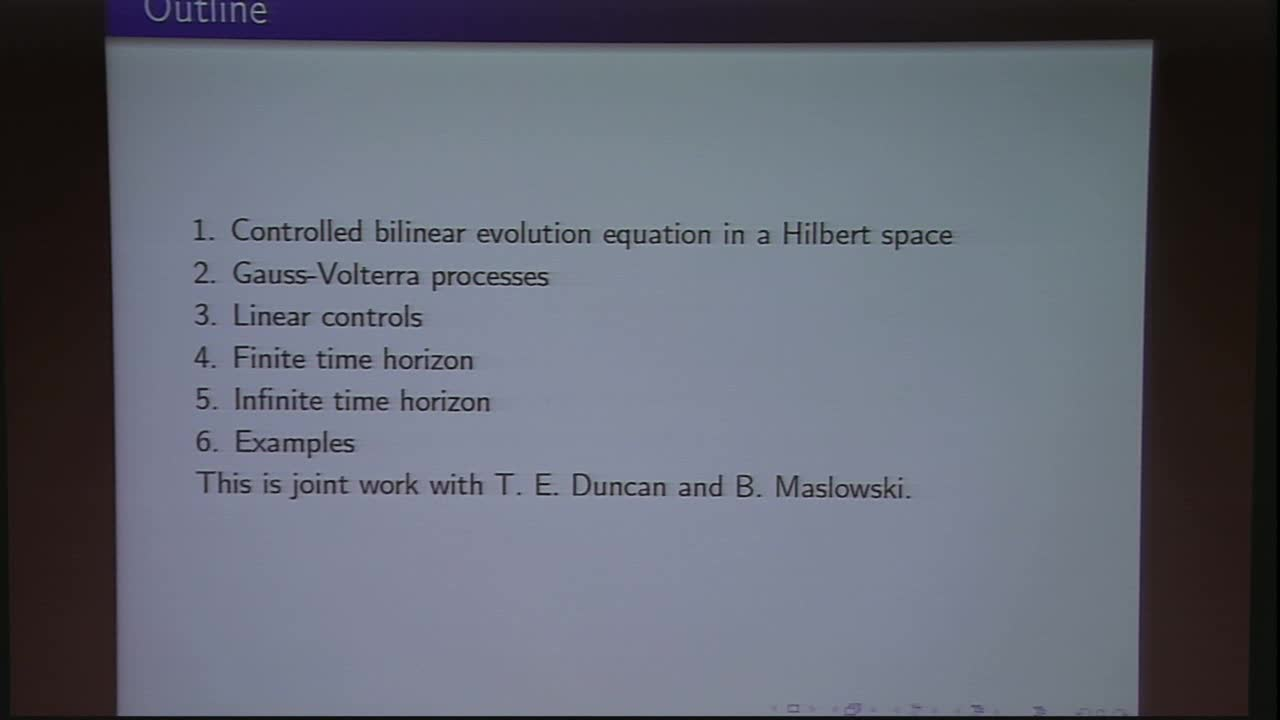## Linear-quadratic control for bilinear evolution equations with Gauss-Volterra processes

May 11, 2018
IMA
Presenters: Bozenna Pasik-Duncan

Watch Video

#### Abstract

Some control problems are explicitly solved for bilinear evolution equations where the noise is a Gauss-Volterra process. The Gauss-Volterra noise processes are obtained from the integral of a Brownian motion with a suitable kernel function. These noise processes include fractional Brownian motions with Hurst parameter from (1/2, 1), Liouville fractional Brownian motions with H from (1/2, 1(, and some multi fractional Brownian motions. The controls are chosen from the family of linear feedback gains. The optimal gain is different from the control problem for a linear equation with a quadratic cost functional. The bilinear equations have drift terms that are linear evolution operators. These equations can model stochastic partial differential equations of parabolic and hyperbolic types. Some examples are given.